# Interleave

On comp.programming mike3 asked for an algorithm to compute "interleave" and "deinterleave" permutations. Here is an implementation in Common Lisp of such an algorithm.

The following diagrams are generated by Graphviz from .dot files generated by interleave.lisp.

2
2 cycles, of lengths: 1, 1.
((1) (0))
2 = 2
0 =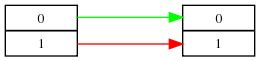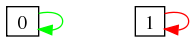4
3 cycles, of lengths: 1, 2, 1.
((3) (2 1) (0))
4 = 22
2 = 2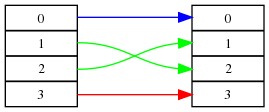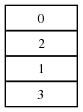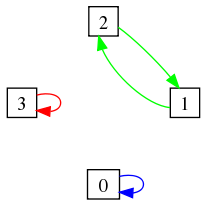6
3 cycles, of lengths: 1, 4, 1.
((5) (2 4 3 1) (0))
6 = 3 * 2
4 = 22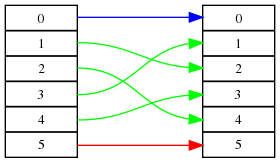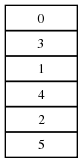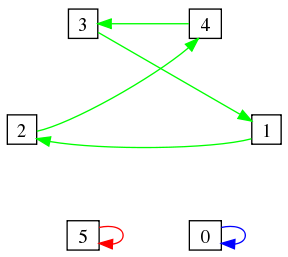8
4 cycles, of lengths: 1, 3, 3, 1.
((7) (6 5 3) (2 4 1) (0))
8 = 23
6 = 3 * 2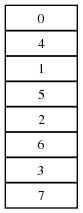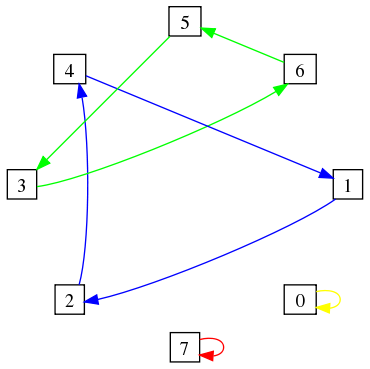10
4 cycles, of lengths: 1, 2, 6, 1.
((9) (6 3) (2 4 8 7 5 1) (0))
10 = 5 * 2
8 = 23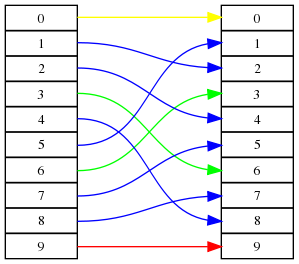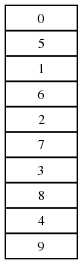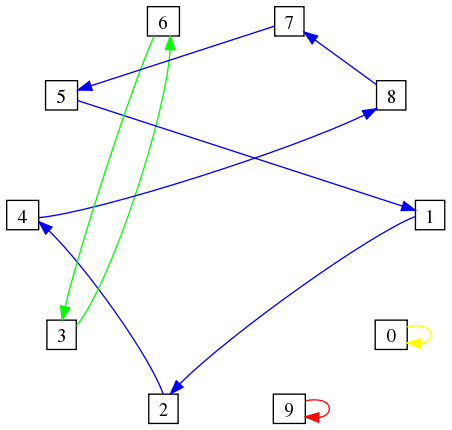12
3 cycles, of lengths: 1, 10, 1.
((11) (2 4 8 5 10 9 7 3 6 1) (0))
12 = 3 * 22
10 = 5 * 2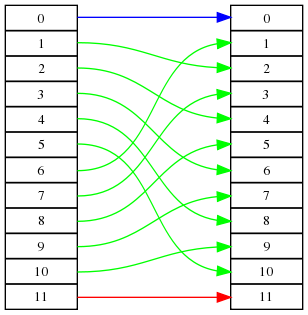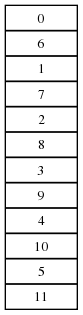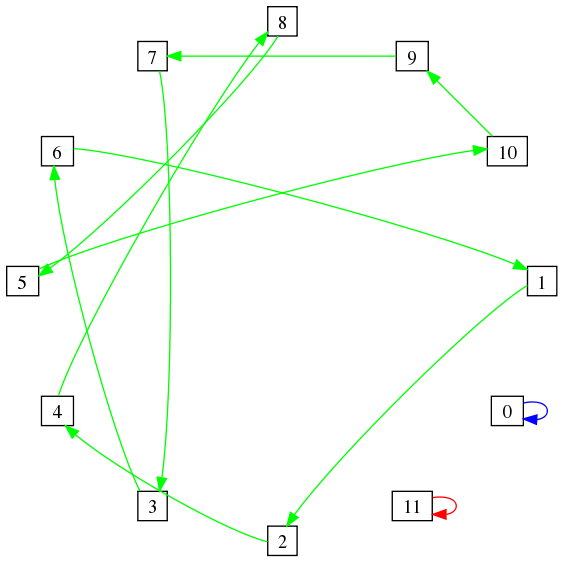14
3 cycles, of lengths: 1, 12, 1.
((13) (2 4 8 3 6 12 11 9 5 10 7 1) (0))
14 = 7 * 2
12 = 3 * 22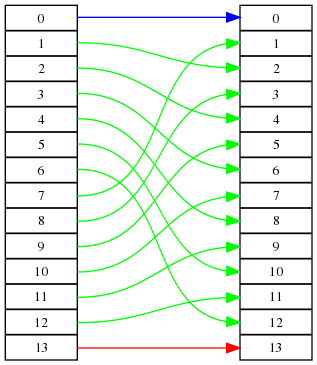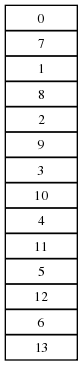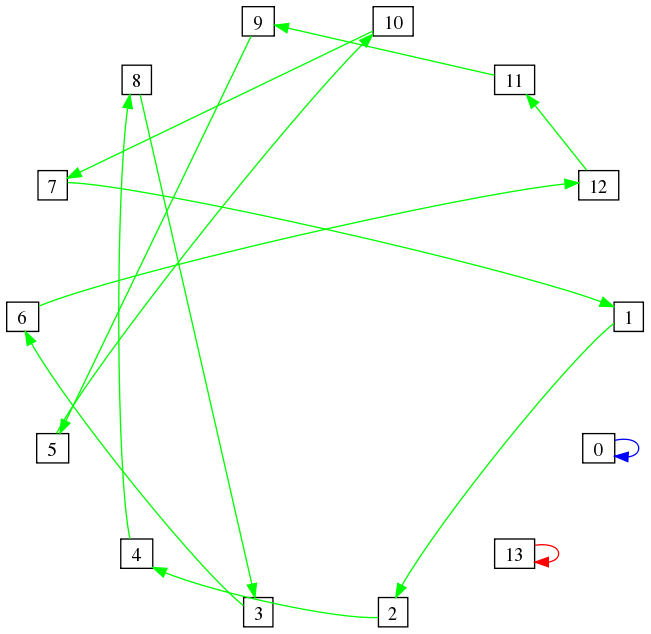``` | Mirror on informatimago.com | Mirror on free.fr | ```